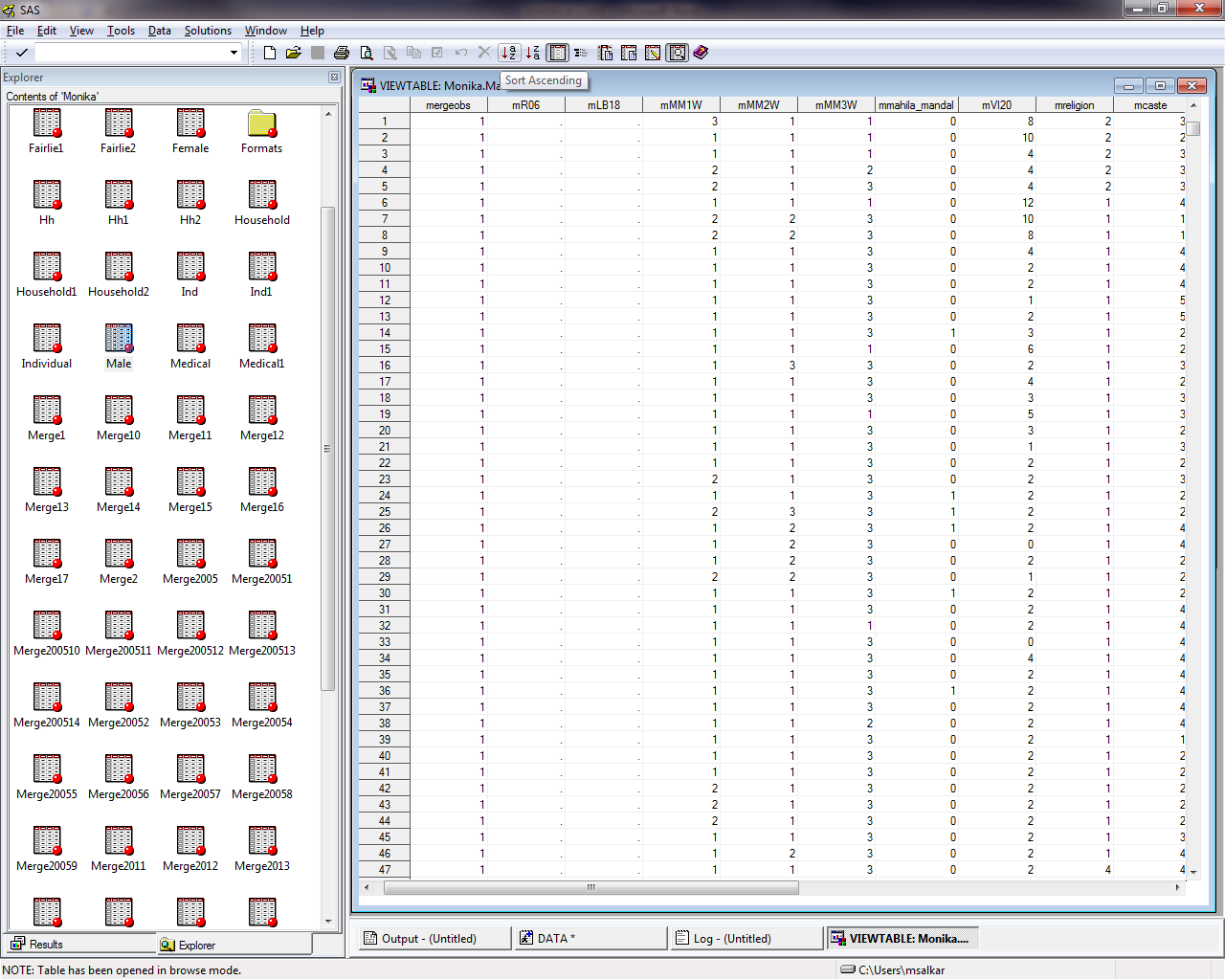## Fairlie decomposiiton method

Hello,

I had post about the Fairlie decomposition method. But again I am having an error, I cannot see the variables RO6 and LB18 in my data set. I tried figuring the problem many times but just cannot figure out what the issue is. I am not getting any error message but just the values of variables are not seen. I am attaching my code as well as the how the dataset looks. Please help. Thank you.

3 REPLIES 3Ksharp
Super User

## Re: Fairlie decomposiiton method

Post your data and code here. Not Excel or Doc file. nobody would like to download them.

or you could post some pictures.

## Re: Fairlie decomposiiton method

This is my code

/*fairlie decomposition 2011-2012*/
libname MONIKA "C:\Users\msalkar\Desktop\THESIS-MONIKA";
options obs=max;
options nolabel ls=75 ps=140;
%let numiterations=100;
%let r=2;
%let gendervars=male female;
%let k=12;

%let definegroup1 = MM1W MM2W MM3W;
%let definegroup2 = mahila_mandal VI20;
%let definegroup3 = religion caste;
%let definegroup4 = BHED INCOME occupation;

%let definegroup6 = RO6  LB18;

%let labelgroup1="media_exposure";
%let labelgroup2="social_events";
%let labelgroup3="cultural_influences";
%let labelgroup4="household_factors";
%let labelgroup5="mothers_age/education";

%let labelgroup6 = "TBA";

%let mdefinegroup1 = mMM1W mMM2W mMM3W;
%let mdefinegroup2 = mmahila_mandal mVI20;
%let mdefinegroup3 = mreligion mcaste;
%let mdefinegroup4 = mBHED mINCOME moccupation;

%let mdefinegroup6 = mRO6  mLB18;

%let wdefinegroup1 = wMM1W wMM2W wMM3W;
%let wdefinegroup2 = wmahila_mandal wVI20;
%let wdefinegroup3 = wreligion wcaste;
%let wdefinegroup4 = wBHED wINCOME woccupation;

%let wdefinegroup6 = wRO6  wLB18;

%let vars= &definegroup1 &definegroup2 &definegroup3 &definegroup4 &definegroup5  &definegroup6;
%let mvars= &mdefinegroup1 &mdefinegroup2 &mdefinegroup3 &mdefinegroup4 &mdefinegroup5  &mdefinegroup6;
%let wvars= &wdefinegroup1 &wdefinegroup2 &wdefinegroup3 &wdefinegroup4 &wdefinegroup5  &wdefinegroup6;

data monika.merge2012;
set monika.merge2011;
mergeobs=1;
y=child_immunization;
if y=. or MM1W=. or MM2W=. or MM3W=. or INCOME=. or FHEADAGE=. or VI20=. or mahila_mandal=. or
religion=. or caste=. or occupation=. or mothers_education=. then delete;
if wt=. or wt<=0 then delete;
run;

data monika.regdata;
set monika.merge2012;
if male=1 or female=1; run;

data monika.male (keep=ym wtm &mvars mergeobs);
set monika.merge2012 ;
array varsa(&k) &vars;
array mvarsa(&k) &mvars;
ym=y;
wtm=wt;
do i=1 to &k;
mvarsa(i)=varsa(i);
end;
if male=1 then output; run;

data monika.female (keep=yw wtw &wvars mergeobs);
set monika.merge2012;
array varsa(&k) &vars;
array wvarsa(&k) &wvars;
yw=y;
wtw=wt;
do i=1 to &k;
wvarsa(i)=varsa(i);
end;
if female=1 then output; run;

After running this I do not get an error but in the data set the values of the variables RO6 and LB18 are not seen. Below is the dataset;Ksharp
Super User

## Re: Fairlie decomposiiton method

```Sorry. I have no time to check all these code. It is too long.
Start a brad new session, post your code too,and let others see this .

```
Discussion stats
• 3 replies
• 676 views
• 0 likes
• 2 in conversation